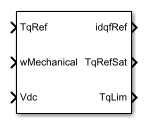# SM Current Reference Generator

Synchronous machine current reference generator

•Libraries:
Simscape / Electrical / Control / SM Control

## Description

The SM Current Reference Generator block implements a current reference generator for synchronous machine (SM) current control in the rotor d-q reference frame.

### Defining Equations

The SM Current Reference Generator block can obtain the current reference using one of these methods:

• Zero d-axis control (ZDAC).

• Lookup tables.

For the ZDAC method, the block sets:

• The d-axis current reference ${i}_{d}^{ref}$ to zero:

`${i}_{d}^{ref}=0,$`

• The field current reference ${i}_{f}^{ref}$ using the torque reference:

`${i}_{f}^{ref}=\frac{|{T}_{ref}{i}_{f,max}|}{{T}_{max}},$`

where if,max is the maximum field current and Tmax is the maximum torque.

• The q-axis current reference ${i}_{q}^{ref}$ using the torque equation:

where Tref is the reference torque input and Kt is the torque constant of the synchronous machine expressed by the simplified torque equation $T={K}_{t}{i}_{f}{i}_{q}$.

For operation below the base speed of the synchronous machine, ZDAC is a suitable method. Above base speed, a field weakening controller is required to adjust the d-axis reference.

To pregenerate the current references for several operating points, define three lookup tables using the lookup tables approach:

`${i}_{d}^{ref}=\text{f}\left({n}_{m},{T}_{ref},{v}_{dc}\right),$`

and

## Ports

### Input

expand all

Desired mechanical torque produced by the synchronous machine.

Data Types: `single` | `double`

Mechanical angular velocity of the synchronous machine rotor, obtained via direct measurement from the synchronous machine.

Data Types: `single` | `double`

DC-link voltage of the converter. For the ZDAC method, this value is used to limit the output reference torque and torque limit. For the lookup table method, this value is used as an input to the lookup tables.

Data Types: `single` | `double`

### Output

expand all

Reference d-q and field currents to be given as inputs to a current controller.

Data Types: `single` | `double`

Reference torque saturated by the calculated torque limit TqLim.

Data Types: `single` | `double`

Torque limit imposed by both the electrical and mechanical constraints of the system.

Data Types: `single` | `double`

## Parameters

expand all

General Parameters

Nominal DC-link voltage of the electrical source.

Maximum synchronous machine power.

Maximum synchronous machine torque.

Maximum field current of the synchronous machine.

Sample time for the block (-1 for inherited). If this block is used inside a triggered subsystem, the sample time should be -1. If this block is used in a continuous variable-step model, then the sample time can be explicitly specified.

Reference Generation Strategy

Select the strategy for determining current references.

Torque constant of the synchronous machine.

Speed vector used in the lookup-tables for determining current references.

Torque vector used in the lookup-tables for determining current references.

DC-link voltage vector used in the lookup-tables for determining current references.

Direct-axis current reference lookup data.